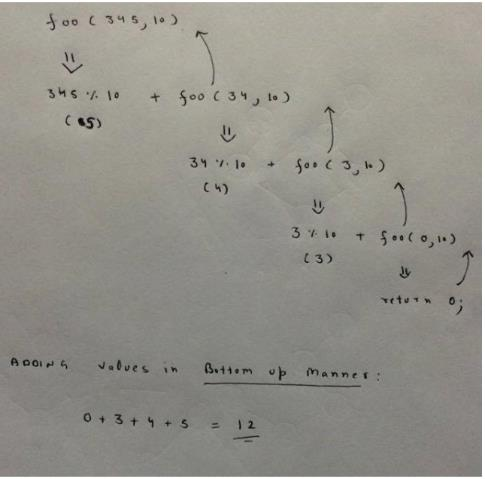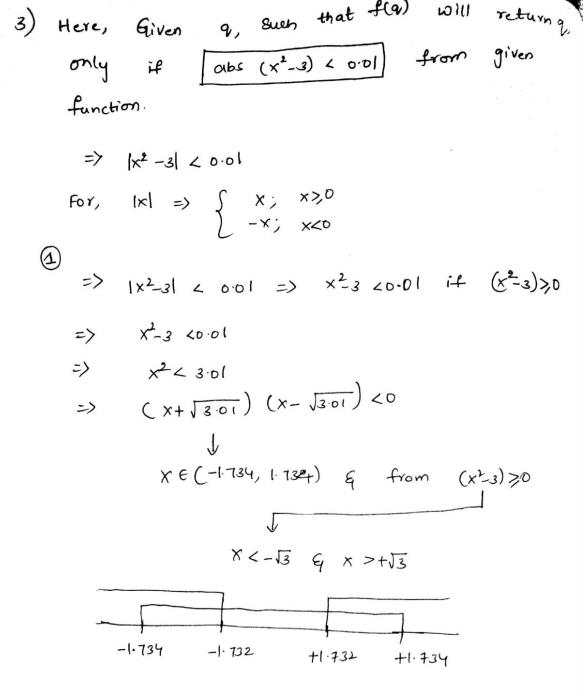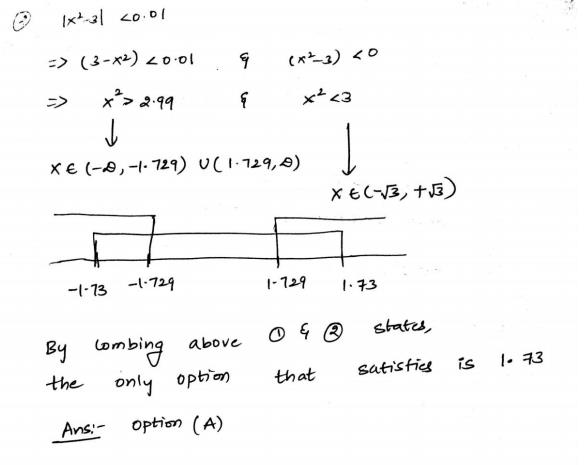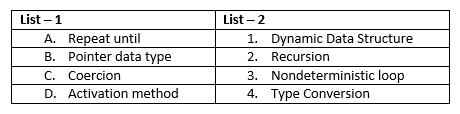Courses

# Test: Recursion- 3

## 20 Questions MCQ Test Programming and Data Structures | Test: Recursion- 3

Description
This mock test of Test: Recursion- 3 for Computer Science Engineering (CSE) helps you for every Computer Science Engineering (CSE) entrance exam. This contains 20 Multiple Choice Questions for Computer Science Engineering (CSE) Test: Recursion- 3 (mcq) to study with solutions a complete question bank. The solved questions answers in this Test: Recursion- 3 quiz give you a good mix of easy questions and tough questions. Computer Science Engineering (CSE) students definitely take this Test: Recursion- 3 exercise for a better result in the exam. You can find other Test: Recursion- 3 extra questions, long questions & short questions for Computer Science Engineering (CSE) on EduRev as well by searching above.
QUESTION: 1

### Consider the following recursive function fun(x, y). What is the value of fun(4, 3) int fun(int x, int y)  {   if (x == 0)     return y;   return fun(x - 1,  x + y);

Solution:

he function fun() calculates and returns ((1 + 2 … + x-1 + x) +y) which is x(x+1)/2 + y.

QUESTION: 2

### Predict output of following program #include <stdio.h> int fun(int n) {     if (n == 4)        return n;     else return 2*fun(n+1); } int main() {    printf("%d ", fun(2));    return 0;

Solution:

Fun(2) = 2 * Fun(3) and Fun(3) = 2 * Fun(4) ....(i)
Fun(4) = 4 ......(ii)
From equation (i) and (ii),
Fun(2) = 2 * 2 * Fun(4)
Fun(2) = 2 * 2 * 4
Fun(2) = 16.

So, C is the correct answer

QUESTION: 3

### What does the following function do? int fun(int x, int y) { if (y == 0)   return 0; return (x + fun(x, y-1));

Solution:

The function adds x to itself y times which is x*y.

QUESTION: 4

What does the following function print for n = 25?

void fun(int n)

{

if (n == 0)

return;

printf("%d", n%2);

fun(n/2);

Solution:

The function mainly prints binary representation in reverse order.

QUESTION: 5

Output of following program?
#include<stdio.h>
void print(int n)
{
if (n > 4000)
return;
printf("%d ", n);
print(2*n);
printf("%d ", n);
}
int main()
{
print(1000);
getchar();
return 0;
}

Solution:

First time n=1000 Then 1000 is printed by first printf function then call print(2*1000) then again print 2000 by printf function then call print(2*2000) and it prints 4000 next time print(4000*2) is called. Here 8000 is greater than 4000 condition becomes true and it return at function(2*4000). Here n=4000 then 4000 will again print through second printf. Similarly print(2*2000) after that n=2000 then 2000 will print and come back at print(2*1000) here n=1000, so print 1000 through second printf. Option (B) is correct.

QUESTION: 6

What does fun2() do in general?
int fun(int x, int y)
{
if (y == 0)   return 0;
return (x + fun(x, y-1));
}
int fun2(int a, int b)
{
if (b == 0) return 1;
return fun(a, fun2(a, b-1));
}

Solution:

The function multiplies x to itself y times which is xy.

QUESTION: 7

Predict the output of following program
#include <stdio.h>
int f(int n)
{
if(n <= 1)
return 1;
if(n%2 == 0)
return f(n/2);
return f(n/2) + f(n/2+1);
}
int main()
{
printf("%d", f(11));
return 0;
}

Solution:

On successive recursion F(11) will be decomposed into F(5) + F(6) -> F(2) + F(3) + F(3) -> F(1) + 2 * [F(1) + F(2)] -> 1 + 2 * [1 + F(1)] -> 1 + 2 * (1 + 1) -> 5. Hence , option D is the correct answer i.e, 5.

QUESTION: 8

Predict the output of following program
#include <stdio.h>
int f(int n)
{
if(n <= 1)
return 1;
if(n%2 == 0)
return f(n/2);
return f(n/2) + f(n/2+1);
}
int main()
{
printf("%d", f(11));
return 0;
}

Solution:

On successive recursion F(11) will be decomposed into F(5) + F(6) -> F(2) + F(3) + F(3) -> F(1) + 2 * [F(1) + F(2)] -> 1 + 2 * [1 + F(1)] -> 1 + 2 * (1 + 1) -> 5. Hence , option D is the correct answer i.e, 5.

QUESTION: 9

What does the following function do?
int fun(unsigned int n)
{
if (n == 0 || n == 1)
return n;
if (n%3 != 0)
return 0;
return fun(n/3);
}

Solution:

Lets solve with example, n = 27 which power of 3. First time if condition is false as n is neither equal to 0 nor equal to 1 then 27%3 = 0. Here, again if condition false because it is equal to 0. Then fun(27/3) will call. Second time if condition is false as n is neither equal to 0 nor equal to 1 then 9%3 = 0. Here again if condition false because it is equal to 0. Then fun (9/3) will call and third time if condition is false as n is neither equal to 0 nor equal to 1 then 3%3 = 0. Here again if condition false because it is equal to 0. Then fun(3/3) will call here n==1 if condition gets true and it return n i.e. 1. Option (B) is correct.

QUESTION: 10

What is the return value of the function foo when it is called as foo(345, 10) ?

Solution:

The call foo(345, 10) returns sum of decimal digits (because r is 10) in the number n. Sum of digits for 345 is 3 + 4 + 5 = 12.QUESTION: 11

Consider the same recursive C function that takes two arguments
unsigned int foo(unsigned int n, unsigned int r) {
if (n  > 0) return (n%r +  foo (n/r, r ));
else return 0;
}

What is the return value of the function foo when it is called as foo(513, 2)?

Solution:

foo(513, 2) will return 1 + foo(256, 2). All subsequent recursive calls (including foo(256, 2)) will return 0 + foo(n/2, 2) except the last call foo(1, 2) . The last call foo(1, 2) returns 1. So, the value returned by foo(513, 2) is 1 + 0 + 0…. + 0 + 1. The function foo(n, 2) basically returns sum of bits (or count of set bits) in the number n.

QUESTION: 12

Output of following program?
#include <stdio.h>
int fun(int n, int *f_p)
{
int t, f;
if (n <= 1)
{
*f_p = 1;
return 1;
}
t = fun(n- 1,f_p);
f = t+ * f_p;
*f_p = t;
return f;
}

int main()
{
int x = 15;
printf (" %d n", fun(5, &x));
return 0;
}

Solution:

Let x is stored at location 2468 i.e. &x = 2468

(dots are use just to ensure alignment)
x = 15
fun(5, 2468)
...{t = fun(4, 2468)
.......{t = fun(3, 2468)
...........{t = fun(2,2468)
...............{t = fun(1, 2468)
...................{// x=1
........................return 1}
................t = 1
................f = 2 //1+1 //since *f_p is x
................x = t = 1
................return 2}
...........t = 2
...........f = 2+1
...........x = t = 2
...........return 3}
........t = 3
........f = 3+2
........x = t = 3
........return 5}
....t = 5
....f = 5+3
....x = t = 5
....return 8}

which implies fun (5,2468) is 8.

QUESTION: 13

#include<stdio.h>
int f(int *a, int n)
{
if(n <= 0) return 0;
else if(*a % 2 == 0) return *a + f(a+1, n-1);
else return *a - f(a+1, n-1);
}

int main()
{
int a[] = {12, 7, 13, 4, 11, 6};
printf("%d", f(a, 6));
getchar();
return 0;
}

Solution:

f() is a recursive function which adds f(a+1, n-1) to *a if *a is even. If *a is odd then f() subtracts f(a+1, n-1) from *a. See below recursion tree for execution of f(a, 6). .
f(add(12), 6) /*Since 12 is first element. a contains address of 12 */
|
|
12 + f(add(7), 5) /* Since 7 is the next element, a+1 contains address of 7 */
|
|
|
|
|
|
|
|
|
|
6 + 0
So, the final returned value is 12 + (7 – (13 – (4 + (11 – (6 + 0))))) = 15

QUESTION: 14

Consider the C function given below.
int f(int j)
{
static int i = 50;
int k;
if (i == j)
{
printf(“something”);
k = f(i);
return 0;
}
else return 0;
}

Which one of the following is TRUE?

Solution:

When j is 50, the function would call itself again and again as neither i nor j is changed inside the recursion.

QUESTION: 15

Consider the C function given below.
int f(int j)
{
static int i = 50;
int k;
if (i == j)
{
printf(“something”);
k = f(i);
return 0;
}
else return 0;
}
Which one of the following is TRUE?

Solution:

When j is 50, the function would call itself again and again as neither i nor j is changed inside the recursion.

QUESTION: 16

Consider the following function
double f(double x){
if (abs(x*x - 3) < 0.01) return x;
else return f(x/2 + 1.5/x);
}
Give a value q (to 2 decimals) such that f(q) will return q:_____.

Solution:QUESTION: 17

Consider the following C function.
int fun (int n)
{
int x=1, k;
if (n==1) return x;
for (k=1; k<n; ++k)
x = x + fun(k) * fun(n – k);
return x;
}
The return value of fun(5) is __________.

Solution:

fun(5) = 1 + fun(1) * fun(4) + fun(2) * fun(3) +
fun(3) * fun(2) + fun(4) * fun(1)
= 1 + 2*[fun(1)*fun(4) + fun(2)*fun(3)]

Substituting fun(1) = 1
= 1 + 2*[fun(4) + fun(2)*fun(3)]

Calculating fun(2), fun(3) and fun(4)
fun(2) = 1 + fun(1)*fun(1) = 1 + 1*1 = 2
fun(3) = 1 + 2*fun(1)*fun(2) = 1 + 2*1*2 = 5
fun(4) = 1 + 2*fun(1)*fun(3) + fun(2)*fun(2)
= 1 + 2*1*5 + 2*2 = 15

Substituting values of fun(2), fun(3) and fun(4)
fun(5) = 1 + 2*[15 + 2*5] = 51

QUESTION: 18

Consider the following recursive C function. If get(6) function is being called in main() then how many times will the get() function be invoked before returning to the main()?
void get (int n)
{
if (n < 1) return;
get(n-1);
get(n-3);
printf("%d", n);
}

Solution:

get(6) [25 Calls]
/      \
[17 Calls] get(5)       get(3) [7 Calls]
/     \
get(4)    get(2)[5 Calls]
/    \
[7 Calls] get(3)  get(1)[3 Calls]
/     \
get(2)   get(0)
/    \
[3 Calls]get(1)  get(-1)
/  \
get(0) get(-2)
We can verify the same by running below program. [sourcecode language="CPP"] # include int count = 0; void get (int n) { count++; if (n < 1) return; get(n-1); get(n-3); } int main() { get(6); printf("%d ", count); } [/sourcecode] Output: 25

QUESTION: 19

The function f is defined as follows:
int f (int n) {
if (n <= 1) return 1;
else if (n % 2  ==  0) return f(n/2);
else return f(3n - 1);
}
Assuming that arbitrarily large integers can be passed as a parameter to the function, consider the following statements.
1. The function f terminates for finitely many different values of n ≥ 1.
ii. The function f terminates for infinitely many different values of n ≥ 1.
iii. The function f does not terminate for finitely many different values of n ≥ 1.
iv. The function f does not terminate for infinitely many different values of n ≥ 1.
Which one of the following options is true of the above?

Solution:

The function terminates for all values having a factor of 2 {(2.x)2==0}
So, (i) is false and (ii) is TRUE.
Let n = 3, it will terminate in 2nd iteration.
Let n=5, it will go like 5 - 14 - 7 - 20 - 10 - 5 – and now it will repeat.
And any number with a factor of 5 and 2, there are infinite recursions possible.
So, (iv) is TRUE and (iii) is false.

QUESTION: 20

Match the pairs in the following questions:Solution:

Correct matching is A – 3, B – 1, C – 4, D – 2 Option (C) is correct.